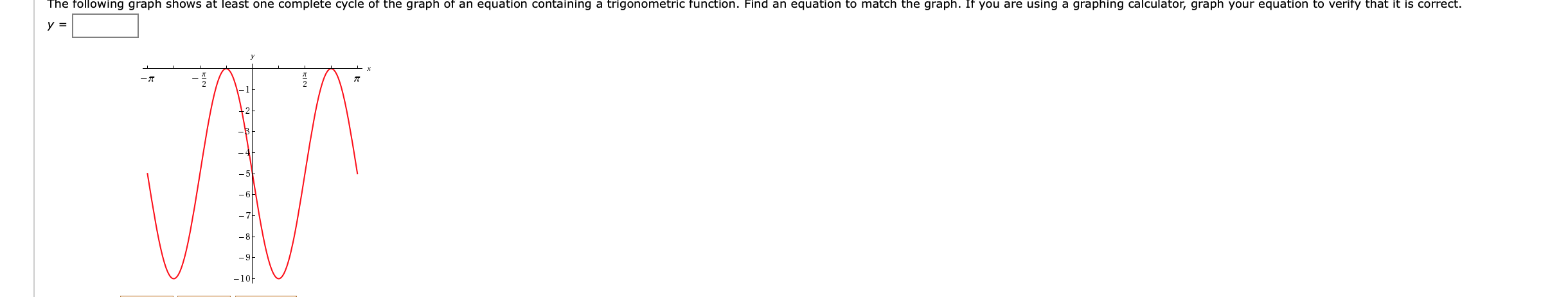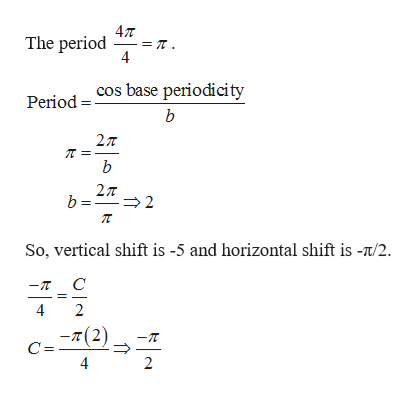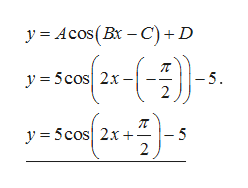The following graph shows at least one complete cycle of the graph of an equation containing a trigonometric function. Find an equation to match the graph. If you are using a graphing calculator, graph your equation to verify that it is correct.y =-A-6-9-10

Questionhelp_outlineImage TranscriptioncloseThe following graph shows at least one complete cycle of the graph of an equation containing a trigonometric function. Find an equation to match the graph. If you are using a graphing calculator, graph your equation to verify that it is correct. y = -A -6 -9 -10 fullscreen
Step 1

Given graph resembles a cosine from π to 3π/4 with amplitude 5.help_outlineImage Transcriptionclose4T The period = T 4 Period COs base periodicity b 27T b 27T b= 2 So, vertical shift is -5 and horizontal shift is -/2 C -T 4 2 -(2) C = 2 fullscreen
Step 2

Now substitute the values of A, B, C and D i...help_outlineImage Transcriptionclosey Acos(Bx-C)+D y = 5cos 2x -5 y5cos 2x - 5 2 fullscreen

Want to see the full answer?

See Solution

Want to see this answer and more?

Our solutions are written by experts, many with advanced degrees, and available 24/7

See Solution
Tagged in

Other